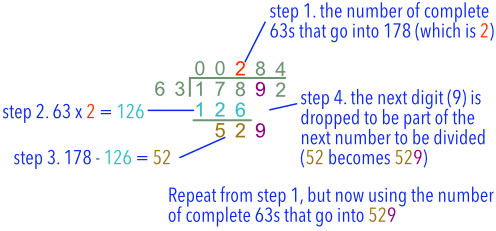Long Division

## Long Division

Long Division is used for larger numbers. It consists of a repeated sequence of four steps:

• Determine how many times a number can be divided;_

• Multiply the divisor by that multiple;

• Set out the remainder;

• Drop the next digit down to create a new number.

Division may not give you an exact answer. Show any remainder as part of the answer.## Example 1

Divide 17892 by 63.

Set out the division as shown below, with the divisor on the left on the left of the bus stop.

Working from left to right, 63 does not go into the first number (1) or the first two numbers (17).

63 divides into 178 twice: place 2 in the answer line (above the 8). Put 2 x 63 (=126) below the 178.  Draw a line below the 126.

Subtract 126 from 178 to obtain 52.  Drop the next digit, 9, down to make a new number from the remainder and the dropped number: 529:

Repeat the steps again until the number has been completely divided.

 0 0 2 8 4 6 3 1 7 8 9 2 1 2 6 5 2 9 5 0 4 2 5 2 2 5 2